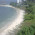Monday, November 23, 2015

Quantum critical spin dynamics of a magnetic impurity in a semiconductor

There is an interesting paper
Quantum critical dynamics of a magnetic impurity in a semiconducting host
Nagamalleswararao Dasari, Swagata Acharya, A. Taraphder, Juana Moreno, Mark Jarrell, N. S. Vidhyadhiraja

The key physics of the Kondo model is the formation of a spin singlet state between the impurity spin and the spins of the electrons in the conduction band. We say, the impurity spin is “screened” by the spins in the conduction band.
The "screening" electrons involved span from the Fermi energy up to some higher energy.
The relevant energy scale is the Kondo temperature which depends in a non-analytic way on the density of states (DOS) at the Fermi energy, and is roughly the binding energy of the spin singlet.
As the DOS goes to zero the Kondo temperature goes to zero.

But, what if there is an energy gap at the Fermi energy, as in a semiconductor?
One might expect that the Kondo effect disappears and the local moment is no longer screened.
Specifically, is there a critical non-zero value of the energy gap below which the Kondo effect survives and one observes at Fermi liquid?
How about if the temperature is larger than the energy gap but less than the Kondo temperature?
Then perhaps the electrons that are thermally excited into the conduction band can screen the impurity spin.

The above fundamental questions are relevant to understanding magnetic semiconductors. They can be addressed by studying the gapped single impurity Anderson model. A number of numerical and analytical studies over the years have produced different answers to the above questions. The current paper gives definitive answers based on state-of-the art Quantum Monte Carlo calculations.

The phase diagram is shown below, with temperature versus the energy gap, delta.
Both are scaled by the Kondo temperature in the absence of the gap. LM denotes an unscreened local moment and GFL a Generalised Fermi Liquid.
The phase diagram is universal in the sense that it is independent of U in the Kondo regime (for large U) and the only relevant energy scale is the Kondo temperature (not the band width or the hybridisation energy).
It is not at all obvious (at least to me) that the universality of the delta=0 case has to extend to the non-zero delta case. But it does.

One sees that the critical value of the energy gap is zero.
Furthermore, above some non-zero temperature, of the order of a fraction of Kondo temperature and about one half of delta, a Generalised Fermi liquid forms where the local moment is completely screened.
The authors also show that the dynamic spin susceptibility associated the spin of impurity exhibits “quantum critical scaling” in the sense that it depends only on omega/T where T is the temperature and omega is the frequency.

Hopefully the paper will stimulate some experiments, either in quantum dots or in semiconductors, to observe this fascinating physics.

1.Can't we understand the universality for delta/T_K by a RG argument ? I am not a expert of Kondo physics, but if I remember correctly, the low-energy behavior of the Kondo problem is governed by an infrared fixed point, which governs the physics for temperature/energy below T_K. Obviously, the gap is a relevant perturbation to the FP, but if it is smaller than T_K, the system will spend enough time at the FP to "forget" about the high-energy physics, hence the universal properties. This seems consistent with both the figure, and the omega/T dependence.

1.Thanks for the interesting and helpful comment.
I think that basically you are right. But, I still find it surprising that the universality extends to energy scales larger than T_K. But, I guess this is also true of the regular Kondo effect.
But, the omega/T dependence goes beyond this since there could be omega/T_K and T/T_K dependence of chi''(omega).

2.Hi Ross,
I think the omega/T dependence is consistent with a QCP scenario. As omega, T and delta are all relevant perturbations to the QCP, one expects a scaling of these variables with an exponent z, i.e. omega s^z, etc. (where s is the rescaling variable). This directly implies that dynamical quantities should scale as omega/T and delta/T, as long as the system is not to far from the QCP (low energy, temperature and gap). This seems consistent with the figures of the paper.

2.Another case is when the metallic host is replaced by a superconductor one, a very similar feature occurs due the superconducting gap in the density of states, this kind of problem was (and yet is) studied in quantum dots systems. Even
in this case, Kondo singlet and superconducting pairing coexist in a
specific range.